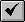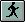HOW TO

# Make Calcapl.ave take NULL shapes into account

Last Published: April 25, 2020

## Summary

Calcapl.ave does not take NULL shapes into account. The NULL shapes get their values from the previous record. The QueryShape request requires that a 'valid' shape object exists first, and in the case of the NULL shapes this is not true; thus, when a value is assigned to the record, it uses the value that already existed in the variable.

## Procedure

1. Open a new script window.

A. Activate the Project window.
B. Click the Scripts icon.
C. Click New.

2. Copy the following code into the new window.

`Code:'-- Name: View.CalculateFeatureGeometry''-- Title:  Calculates feature geometry values'        '-- Topics:  GeoData''-- Description: This is a modified version of calcapl.ave script.'-- It calculates area and perimeter for polygon themes and '-- length for line themes. If the view has been projected the '-- calculations are in projected meters. Otherwise the calculations '-- are in native map units. Modify the script to provide'-- calculation in the current report units of the view.'-- The script processes the list of active themes to calculate'-- area and perimeter, or length, depending on the theme type.''-- The script will add the fields: Area and Perimeter to polygon'-- themes. Length to line themes if they do not exist. If the fields'-- exist their values will be recalculated. Rerun the '-- script if you change the projection of the view.''-- Requires: A view with at least one active theme. You must have '-- write access to the active theme(s).'-- Get the view and its projection if any.theView = av.GetActiveDocthePrj = theView.GetProjectionif (thePrj.IsNull) then  hasPrj = falseelse  hasPrj = trueend'-- Get the list of active themes. if there aren't any,'-- let the user know and exit.theActivethemeList = theView.GetActivethemesif (theActivethemeList.Count = 0) then  MsgBox.Error("No active themes.","")  Exitend'-- Loop through the list of active themes. if you can't'-- edit the theme inform the user.For Each thetheme in theActivethemeList  theFTab = thetheme.GetFTab  if (theFTab.CanEdit.Not) then    MsgBox.Info("Cannot edit table for theme:"++thetheme.AsString,"")    Continue  end  '-- Make the FTAB editable and find out the type of feature it is.  theFTab.SetEditable(TRUE)  theType = theFTab.FindField("shape").GetType  if (theType = #FIELD_SHAPEPOLY) then    '-- If it's polygonal check for the existence of the fields    '-- Area and Perimeter. if they do not exist, create them.    if (theFTab.FindField("Area") = nil) then      theAreaField = Field.Make("Area",#FIELD_DOUBLE,16,3)      theFTab.AddFields({theAreaField})    else      ok = MsgBox.YesNo("Update Area?", "Calculate", true)      if (ok.Not) then        continue      end      theAreaField = theFTab.FindField("Area")    end    if (theFTab.FindField("Perimeter") = nil) then      thePerimeterField = Field.Make("Perimeter",#FIELD_DOUBLE,16,3)      theFTab.AddFields({thePerimeterField})    else      ok = MsgBox.YesNo("Update Perimeter?", "Calculate", true)      if (ok.Not) then        continue      end      thePerimeterField = theFTab.FindField("Perimeter")    end        '-- Loop through the FTAB and find the projected area    '-- and perimeter of each  shape and set the field values    '-- appropriately.    For Each rec in theFTab      theShape = theFTab.ReturnValue(theFTab.FindField("shape"),rec)                if(theShape.isNull) then        theArea = 0.0        thePerimeter = 0.0              else             theFTab.QueryShape(rec,thePrj,theShape)          theArea = theShape.ReturnArea        thePerimeter = theShape.ReturnLength      end            theFTab.SetValue(theAreaField,rec,theArea)      theFTab.SetValue(thePerimeterField,rec,thePerimeter)    end      elseif (theType = #FIELD_SHAPELINE) then    '-- If the data source is linear, check for the existence    '-- of the field "Length". if it doesn't exist, create it.    if (theFTab.FindField("Length") = nil) then      theLengthField = Field.Make("Length",#FIELD_DOUBLE,16,3)      theFTab.AddFields({theLengthField})    else      ok = MsgBox.YesNo("Update Length?", "Calculate", true)      if (ok.Not) then        continue      end      theLengthField = theFTab.FindField("Length")    end         '-- Loop through the FTAB and find the projected length of    '-- each shape and set the field values appropriately.     For Each rec in theFTab      theShape = theFTab.ReturnValue(theFTab.FindField("shape"),rec)      if(theShape.isNull) then        theLength = 0      else                         theFTab.QueryShape(rec,thePrj,theShape)        theLength = theShape.ReturnLength      end      theFTab.SetValue(theLengthField,rec,theLength)    end      end   theFTab.SetEditable(FALSE)end`

3. Click the Compile button.4. Click the Run button.Article ID:000004322

Software:
• Legacy Products

Get help from ArcGIS experts

Contact technical support